메뉴 건너뛰기

#8. Fibonacci Retracement Method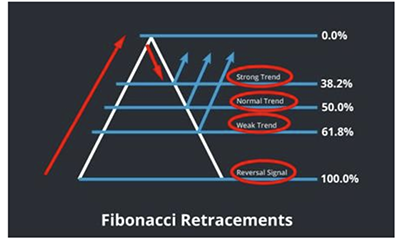What is Fibonacci Retracement?

It is an array of numbers created by Italian mathematician Fibonacci, which is a kind of golden ratio concept.

cf. Fibonacci Retracement [Related]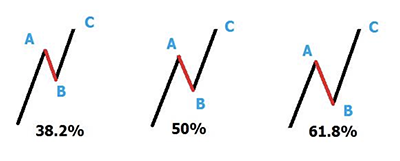The Fibonacci retracement arrangement we usually see is

38.2% / 50% / 61.8%

You can remember about 3 of theseThis Fibonacci retracement is used to predict a rebound or correction period.If you look at the image above, if you see the lowest low of the candle as 0, the highest as 1, and plot this with the Fibonacci scale,

Rebound to 38.2% and correction at 38.2%

Buying Timing: When the rebound starts

Selling Timing: When the correction begins

Easy, right?

Look at the image below and think in your head to understand.Adjust after rebounding to 0.382 sectionAfter about 0.5 section, it rises again0.618 section resistanceAct as a support line after breaking through each section

Summary

A retracement (rebound or correction) may come in the 38.2% / 61.8% ratio range.

Buy when the rebound starts and sell when the correction starts.

 2021년 해외코인거래소 순위 및 추천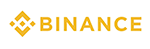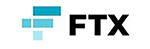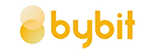거래량 11조(1위) 3.5조 9.5조 레버리지 최대100배 최대100배 최대125배 수수료 - 지정가 : 0.02%           - 시장가 : 0.04%(1위) - 지정가 : -0.025%                - 시장가 : 0.075% - 지정가 : -0.025%                  - 시장가 : 0.075% 거래방법 현물+선물+마진 선물+주식+FOREX 선물 회원가입 회원가입 회원가입 회원가입 할인코드 20% 할인 5% 할인 20% 할인